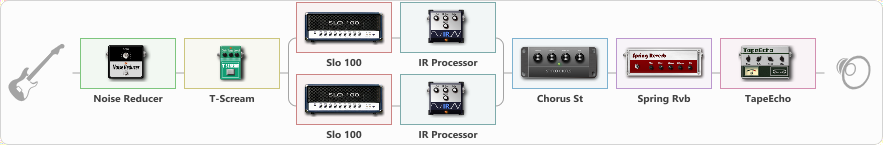# dual metal

Discussion in 'ToneLib-GFX presets' started by Saitama, May 1, 2020.

1. dual metal

Preset name: dual2

Effects chain:Effect: "Noise Reducer" (Dynamics / Filter), active - "yes"
{
"Sens" = 80
"Mode" = Soft
}

Effect: "T-Scream" (Overdrive / Distortion), active - "yes"
{
"Drive" = 0
"Tone" = 65
"Level" = 63
}

Effect: "Splitter" (Dynamics / Filter), active - "yes"
{
"A-Bypass" = Off
"A-Pan" = 0
"A-Level" = 55
"B-Bypass" = Off
"B-Pan" = 0
"B-Level" = 55

'A' branch:
{

Effect: "Slo 100" (Amp simulators), active - "yes"
{
"Gain" = 42
"Bass" = 39
"Middle" = 58
"Treble" = 70
"Presence" = 50
"Master" = 70
"Level (dB)" = 5
}

Effect: "IR Processor" (Cabinets), active - "yes"
{
"IR" = IR_HardSound
"Low Cut (Hz)" = 0
"Hi Cut (kHz)" = 20.0
"Mix" = 100
"Level (dB)" = 0
}
}
'B' branch:
{

Effect: "Slo 100" (Amp simulators), active - "yes"
{
"Gain" = 40
"Bass" = 39
"Middle" = 55
"Treble" = 55
"Presence" = 40
"Master" = 70
"Level (dB)" = 5
}

Effect: "IR Processor" (Cabinets), active - "yes"
{
"IR" = s-preshigh
"Low Cut (Hz)" = 0
"Hi Cut (kHz)" = 20.0
"Mix" = 100
"Level (dB)" = 0
}
}
}

Effect: "Chorus St" (Modulation / Sfx), active - "yes"
{
"Speed" = 1.9
"Depth" = 31
"Center" = 4.4
"Mix" = 40
}

Effect: "Spring Rvb" (Reverb), active - "yes"
{
"Time" = 5.7
"PreDelay" = 0
"LoDamp" = 16
"HiDamp" = 26
"Mix" = 20
}

Effect: "TapeEcho" (Delay), active - "yes"
{
"Time" = 350
"Feedback" = 38
"Tone" = 65
"LoDamp" = 19
"Mix" = 20
}

Note: You will need to download and install the ToneLib-GFX software to use the preset.

#### Attached Files:

• ###### dual_metal.tlgfx
File size:
4.7 KB
Views:
2,795
ARSZ, HeavenlDevil, jes and 4 others like this.